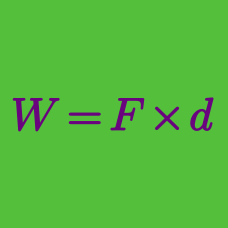Classical Mechanics

# Rocket physics

A rocket's engine ejects fuel at a rate of $40 \text{ kg/s}$ with an exhaust velocity of $4000 \text{ m/s}.$ If the rocket's initial mass is $24000 \text{ kg},$ what is the approximate change in velocity after $24$ seconds?

A rocket whose mass is $M_i = 2600 \text{ kg},$ of which $2080 \text{ kg}$ is fuel, consumes fuel at a rate of $R = 26 \text{ kg/s}.$ The exhaust velocity of the fuel relative to the rocket engine is $v_e = 400 \text{ m/s}.$ If the fuel consumption rate is changed to double the thrust, how long can the rocket fire the engine?

A $5000 \text{ kg}$ space shuttle moving toward Moon with velocity $100 \text{ m/s}$ fires its rocket engine. The rocket engine ejects exhaust at a speed of $300 \text{ m/s}$ relative to engine. If the final speed of the shuttle is $130 \text{ m/s},$ what is the approximate amount of exhaust the shuttle has ejected?

A rocket of mass $5000 \text{ kg}$ ejects fuel at a rate of $R = 5 \text{ kg/s}$ with an exhaust velocity of $v_e = 3000 \text{ m/s}.$ If the rocket's initial velocity is $v_i = 1800 \text{ m/s},$ approximately how long will it take to reach a velocity of $v_f =2700 \text{ m/s}?$

A rocket whose initial mass is $M_i = 1380 \text{ kg}$ consumes fuel at rate of $R = 22 \text{ kg/s}.$ The exhaust velocity of fuel relative to the rocket engine is $v_e = 200 \text{ m/s}.$ Find the thrust that the rocket engine provides.

×